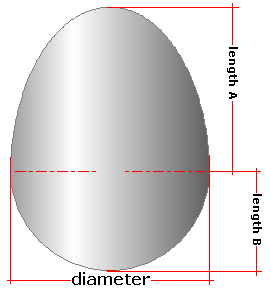Spike's Calculators

# Egg-Shaped Tank - Three MeasurementsThis calculator requires three measurements to calculate a tank's volume, capacity and surface area in the shape of an egg. This calculator uses inches for measurements, requiring the diameter at the widest part of the egg shape, plus two height measurements from above and below this point. The measurements needed are in inches.

##### Approximate weight of Fuel or Water
```one US gallon of water = 8.34 pounds.
one US gallon of diesel fuel = 7.1 pounds
one US gallon of gas = 6 pounds
```

### Egg Shaped Tank

Upper Length A in
Lower Length B in
Diameter in
Liquid Weight-Pounds per US Gallon lb/gal US

#### Results:

###### Tank Volume:
In Cubic Inches in³
In Cubic Feet ft³
In Cubic Metres
###### Tank Capacity:
In US Gallons gal US
In Imperial Gallons gal Imperial
In Litres L
###### Liquid Weight and Surface Area
Weight of Liquid lbs
Surface Area in Square Inches in²
In Square Feet ft²
In Square Millimetres mm²
In Square Metres

#### Calculator

1. enter the length A in inches
2. the length B in inches
3. the diameter of the tank in inches
4. the weight of the liquid in pounds per US gallon

#### Results

1. the volume of the tank in cubic inches
2. the volume in cubic feet
3. the volume in cubic metres
4. the tank capacity in US gallons
5. the capacity in Imperial gallons
6. the capacity in litres
7. full tank liquid weight based on lbs/gal US
8. the surface area of the tank in square inches
9. the surface area in square feet
10. the surface area in square millimetres
11. the surface area in square metres
##### Formula
```V = 2/3𝝅(D/2)²H
where V is the volume
𝝅 = 3.14159265
D the diameter of the egg tank on its widest point
H the height of the egg
```
##### Conversions
```one cubic inch (in³) = 0.000578703703703704 cubic feet (ft³)
one cubic inch (in³) = 0.000016387064 cubic metres (m³)
one cubic inch (in³) = 0.00432900432900432 gallons (gal) US
one cubic inch (in³) = 0.00360465014990905 gallons (gal) Imperial
one cubic inch (in³) = 0.016387064 litres (L)
```

### Egg Shape